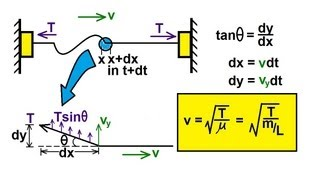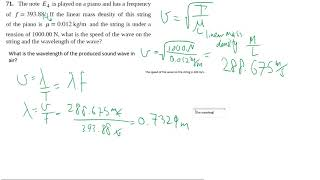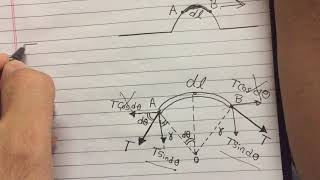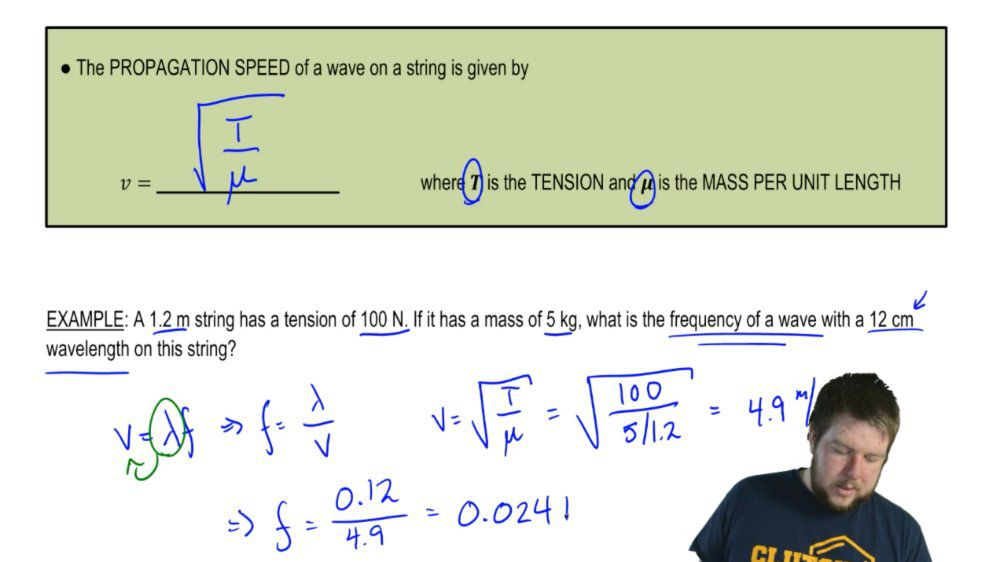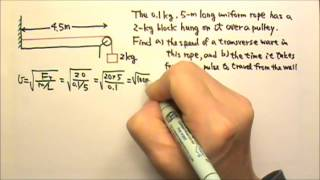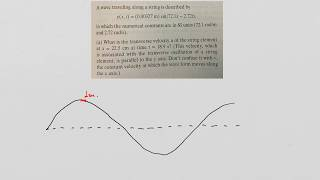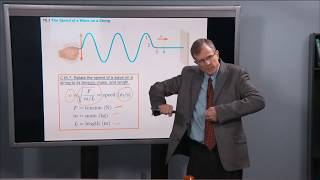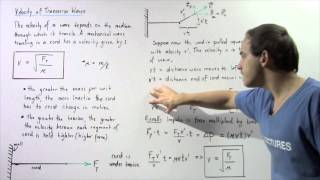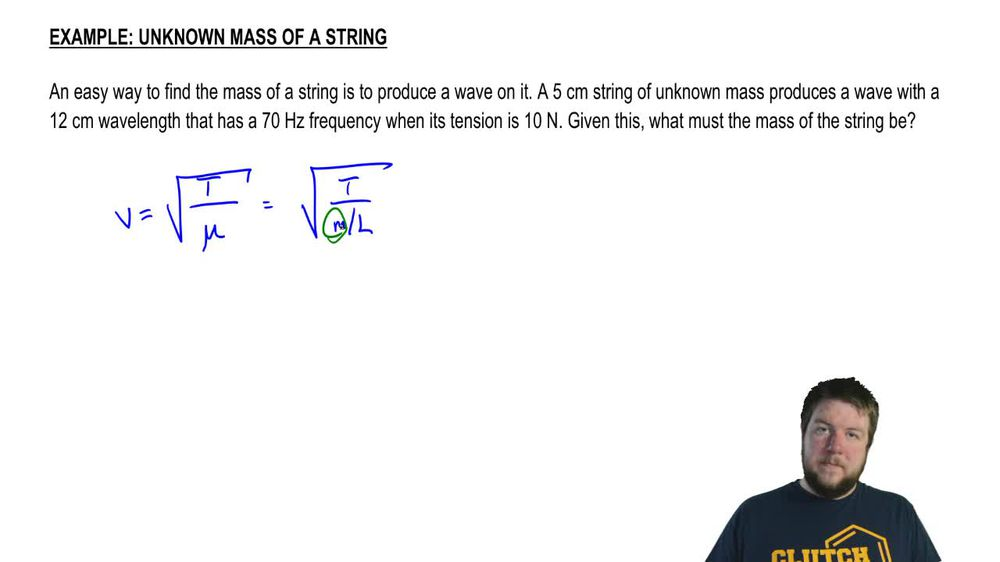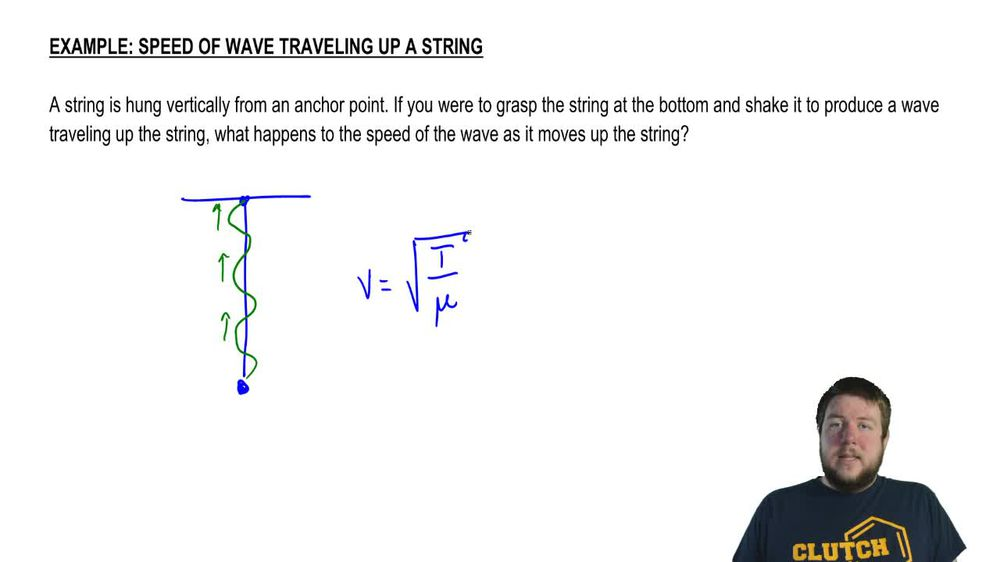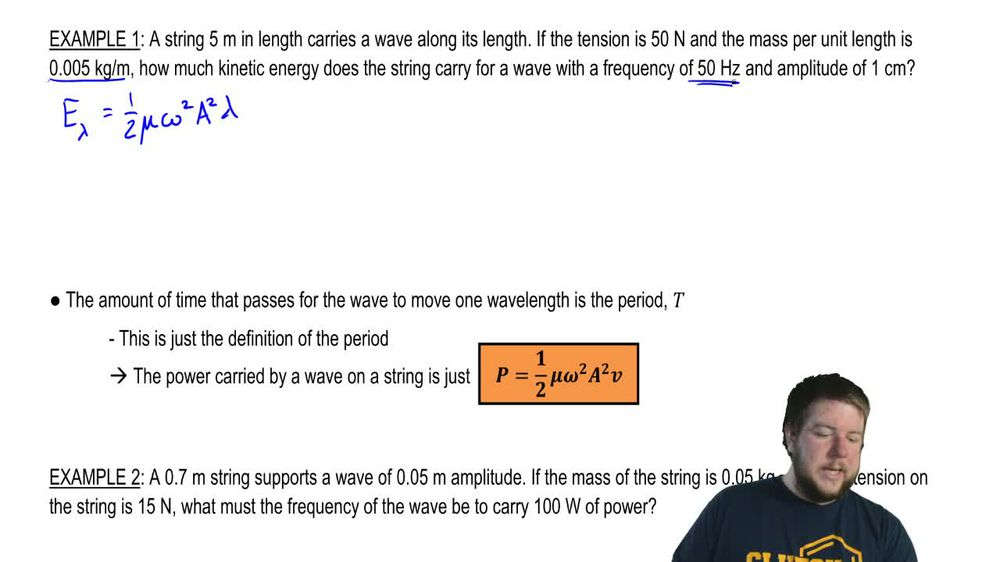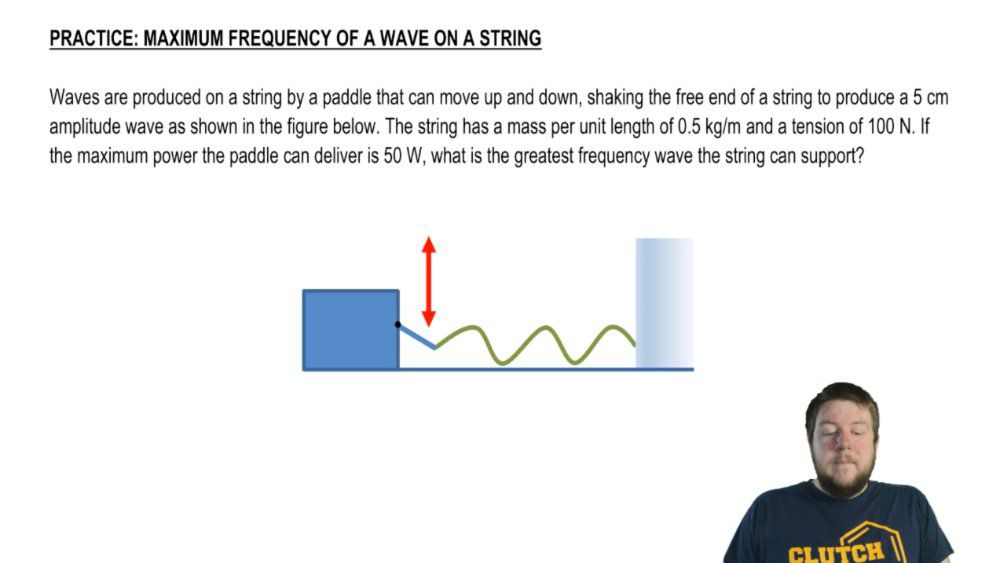Start typing, then use the up and down arrows to select an option from the list.
1. 18. Waves & Sound2. Velocity of Transverse Waves (Strings)# Unknown Mass Of A String

by Patrick Ford
120 views
2
0
Hey, guys, let's do a quick example. An easy way to find the mass of a string is to produce a wave on it. A five centimeter string off unknown mass produces a wave with a centimeter wavelength that has a 70 hertz frequency. When it's tension is 10 Newtons Given this, what must the mass of the spring be? Sorry. The string. Be okay? Well, we're the only equation that we have so far that deals with the mass. On a string is the speed equation. Speed of waves on a string are given by the spirit of the tension divided by the mass per unit length. Okay, this is the tension divided by the mass per unit length. What we want is toe isolate that mass. Okay, so we can just rewrite This is the square root. Sorry. This squared of the speed is t over em. Over. L take em over. Ellen, multiply into the numerator. Take V squared divided into the denominator. This is M over. L is t over v squared And then lastly take l multiply it into the numerator and we have em. Is t l over b squared. So what? We need to know is V squared. Well, the relationship for speed off any wave is that it's equal to the wavelength times the frequency. We know that it's a 12 centimeter wavelength and a 70 hertz frequency. So this is gonna be a 0.12 You wanna in meters times 70 which is 84 m per second. Now we console for the mass, so the mass is gonna be t l over V squared. The tension is 10 Newtons. The length is five centimeters or m. And the speed we found was 84 and we that squared. And that comes out to 0007 kg. Or if I reduce that by a factor of 1000 that's 7 g. Either of these answers are perfectly fine. Alright, guys, that wraps up this problem. Thanks for watching